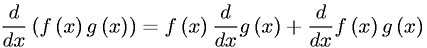Equations > Calculus > Differentiation/Differentals > Product Rule of Differentiation

### Product Rule of DifferentiationLatex Code:

MathML Code:

 $\fracd\mathrm{dx}\left(f\left(x\right)g\left(x\right)\right)=f\left(x\right)\fracd\mathrm{dx}g\left(x\right)+\fracd\mathrm{dx}f\left(x\right)g\left(x\right)$

MathType 5.0: